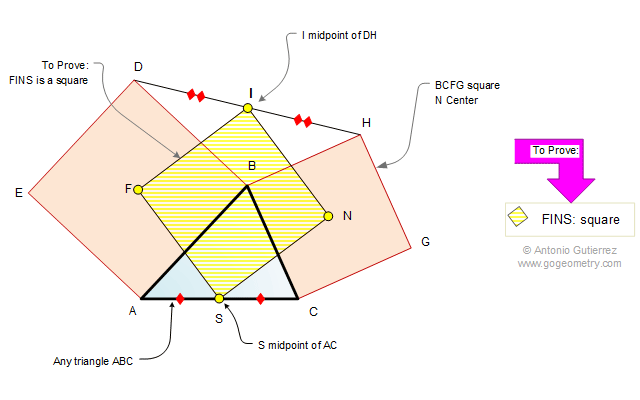Problem 501. Finsler-Hadwiger theorem. Triangle, Two Squares, Center, Midpoint, another Square. Level: High School, College, SAT Prep. The drawing shows a triangle ABC with the squares ABDE and BCGH of centers F and N, respectively. If S and I are the midpoints of AC and DH, respectively, prove that FINS is another square.See also: Artwork of problem 501 Recent Additions